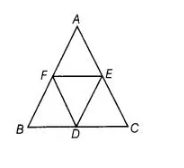# D, E and F are the mid-points of the sides BC,`
Question:

D, E and F are the mid-points of the sides BC, CA and AB, respectively of an equilateral ΔABC. Show that ΔDEF is also an equilateral triangle.

Solution:

Given In equilateral ΔABC, D, E and F are the mid-points of sides BC, CA and AB, respectively.

To show ΔDEF is an equilateral triangle.Proof Since in ΔABC, E and F are the mid-points of AC and AB respectively, then EF || BC and

EF =½ BC ,,.(i)

DF || AC, DE || AB

DE = ½ AB and FD = ½ AC [by mid-point theorem]… (ii)

since ΔABC is an equilateral triangle

AB = BC = CA

=> ½ AB = ½ BC = ½ CA  [dividing by 2]

=> ∴ DE = EF = FD   [from Eqs. (i) and (ii)]

Thus, all sides of ADEF are equal.

Hence, ΔDEF is an equilateral triangle.

Hence proved.